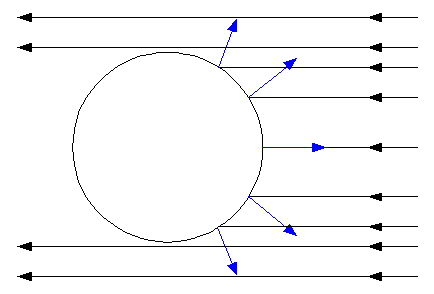# A. P. French homework 1-2(a) Force exerted on satellite by Sun's radiation



## Homework Statement

The intensity of the Sun's radiation just outside the Earth's atmosphere is approximately $8 \cdot 10^4 \frac {joules}{m^2 \cdot min}$. Echo II is a spherical shell of radius $r_0 =$ 20.4m. Its skin consists of a layer of Mylar plastic ... between two layers of aluminum. ... Aluminum's reflection coefficient is .81.

Approximately what force does [the Sun's] radiation exert on the Echo II reflecting satellite balloon?

## Homework Equations

(From experiment explained earlier in the chapter)

$$c = \frac {W(1 + ρ)}{F}$$

(Solved for force)

$$F = \frac {W(1 + ρ)}{c}$$

Where W is the rate of arrival of energy, F is the rate of change of momentum (force), ρ is the reflection coefficient, and c is the speed of light in a vacuum.

## The Attempt at a Solution

This seemed like a flux problem, so

$$\iint_D{F(r(\varphi,\vartheta)) \cdot (r_\varphi \times r_\vartheta) dA}$$

Where

$$r(\varphi,\vartheta) = r_o sin \varphi cos \vartheta \textbf{i} + r_o sin \varphi sin \vartheta \textbf{j} + r_o cos \varphi \textbf{k}$$

$$F = -\frac{W(1 + ρ)}{c}\textbf{k}$$

$$r_\varphi \times r_\vartheta = r_o^2 sin^2 \varphi cos \vartheta \textbf{i} + r_o^2 sin^2 \varphi sin \vartheta \textbf{j} + r_o^2 sin \varphi cos \varphi \textbf{k}$$

so

$$F(r(\varphi,\vartheta)) \cdot (r_\varphi \times r_\vartheta) = -\frac{W (1 + ρ)}{c} r_o^2 sin \varphi cos \varphi \textbf{k}$$

$$-\frac{W (1 + ρ)}{c} r_0^2 \int_0^{2\pi}{\int_0^\frac{\pi}{2}{sin \varphi cos \varphi d\varphi d\vartheta}}$$

my problem : I get .0105 N when the back of the book solution is .00576 N

Did I set something up wrong?

Last edited:

## Answers and Replies

Simon Bridge
Science Advisor
Homework Helper
W=intensity x cross-sectional area = 1.05e8J/min = 1.74e6 J/s
I get F = 0.0105N too.

It is 0.00576 ≈ W/c which would suggest a reflectivity of 0...

Thanks for your reply. You mentioned W/c ≈ .00576 but I get the wrong dimensions (pressure in Pascals) when I do that. Doing W$\pi r^2$ / c gets me .00581 which seems a bit too much error compared to the expected solution...

Simon Bridge
Science Advisor
Homework Helper
You forgot the dimensions of reflectivity... just 'cause it's zero don't mean it don't have dimensions :)

If you divide our result by the books you get: 1.8070 (4dp) ... since 1+ρ=1.81 it is tempting to imagine that the provided value of ρ=0.81 may be rounded up from 0.807 leading to the ratio.

But I agree - unless you can think of some reason the satellite reflects nothing, this is not the reason for the discrepancy.

Either the relation is incorrect or there is another factor not included.
(Or the book in incorrect on this one...)
Back to the drawing board.

Hi Simon. The reflectivity is a dimensionless number in the book. I imagined it was like a coefficient of friction. Your suggestion that the reflectivity is rounded is interesting because the table with the coefficients only lists them to two significant figures. Still, it's hard to believe that the book would make such an important and simple error. Data for the mass of the satellite is given, but I assumed it was for part b, which asks for the force of gravity between the satellite and the sun.

gneill
Mentor
Have you considered that for a spherical surface, the reflected light is not all perpendicular to its incoming direction? Away from the sub-solar point on the sphere's surface, the net force per unit area along the Sun-satellite direction will diminish, reaching zero at the terminator.#### Attachments

Last edited:
Simon Bridge
Science Advisor
Homework Helper
Argh - the total flux intercepted is in the crossection, but the force in each dS is not all in the same direction!!!!

The integral may be easiest to do in cylindrical coords with z in the flux direction ... only the component of the force normal to the surface counts - and the coordinate-radial components of that cancels. I'm too tired to check it :(